PRICE-SALES MULTIPLES

Issues in using price-sales multiples

• Unlike price-earnings and price-book value ratios, which can become negative and not meaningful, the price-sales multiple is available even for the most troubled firms.
• Unlike earnings and book value, which are heavily influenced by accounting decisions on depreciation, inventory and extraordinary charges, revenue is relatively difficult to manipulate.
• Price-sales multiples are not as volatile as price-earnings multiples, and hence may be more reliable for use in valuation.
• The price-sales multiple provides a convenient handle for examining the effects of changes in pricing policy and other corporate strategic decisions.

Price-Sales Ratios and Fundamentals

PS Ratio for a stable firm

The value of equity for a stable firm, using the Gordon growth model is: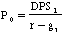Defining the Profit Margin = EPS0 / Sales per share, the value of equity can be written as: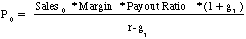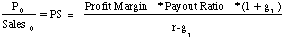If the profit margin is based upon expected earnings in the next time period, this can be simplified to,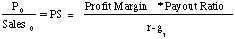Illustration 20: Estimating the PS ratio for a stable firm: International Multifoods

International Multifoods had revenues per share of \$134.70, earnings per share of \$1.50 in 1994, and paid out 55% of its earnings as dividends that year. The growth rate in earnings and dividends, in the long term, is expected to be 6%. The net profit margin at International Multifoods in 1992 was 1.1%. The beta for International Multifoods is 0.80 and the treasury bond rate is 7.5%.

Current Dividend Payout Ratio = 55%

Expected Growth Rate in Earnings and Dividends = 6%

Net Profit Margin = Net Income/Revenues = \$1.50/134.70 = 1.11%

Cost of Equity = 7.5% + 0.8*5.5% =11.90%

PS Ratio based on fundamentals = 0.011 * 0.55 *1.06 / (.119 -.06) = .1097

International Multifoods was selling at a PS ratio of 0.14 at the time of this analysis.

PS Ratio for a high growth firm

When the growth rate is assumed to be constant forever, the dividend discount model can be written as follows: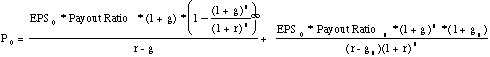where,

g = Growth rate in the first n years

Payout = Payout ratio in the first n years

gn = Growth rate after n years forever (Stable growth rate)

Payoutn = Payout ratio after n years for the stable firm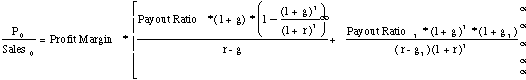The left hand side of the equation is the price-sales ratio. It is determined by--

(a) Net Profit Margin: Earning per share / Revenue per share. The price-sales ratio is an increasing function of the net profit margin.

(b) Payout ratio during the high growth period and in the stable period: The PS ratio increases as the payout ratio increases.

(c) Riskiness (through the discount rate r): The PS ratio becomes lower as riskiness increases.

(d) Expected growth rate in Earnings, in both the high growth and stable phases: The PS increases as the growth rate increases, in either period.

This formula is general enough to be applied to any firm, even one that is not paying dividends right now.

Illustration 21: Estimating the PS ratio for a high growth firm in the two-stage model

Assume that you have been asked to estimate the PS ratio for a firm which has the following characteristics:

Growth rate in first five years = 20% Payout ratio in first five years = 20%

Growth rate after five years = 8% Payout ratio after five years = 68%

Beta = 1.0 Riskfree rate = T.Bond Rate = 6%

Net Profit Margin = 10%

Required rate of return = 6% + 1(5.5%)= 11.5%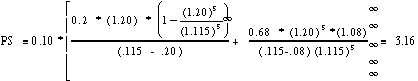The estimated PS ratio for this firm is 3.16.

Illustration 22: Estimating the price-sales multiple for Marks & Spencer

High Growth Period

Expected length of high growth period = 10 years (Longer because of international growth prospects)

Expected growth rate during high growth period = 16.00%

Net Profit Margin during high growth period = Net Profits / Revenues = 8.88% (Same as 1994)

Payout Ratio during high growth period = 44.29% (Same as 1994)

Beta during high growth period = 0.99 (Expected to remain unchanged)

Stable Growth Period

Expected growth rate during stable growth period = 6.00%

Expected Payout Ratio during stable growth period = 65.00%

Beta is expected to remain unchanged.

The long term gilt rate (government bond) in the UK was 8.50% at the time of this analysis, and a risk premium of 5.50% was used.

Cost of Equity = 8.5% + 0.99 (5.5%) = 13.95%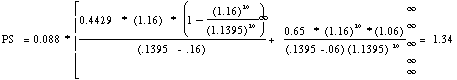The market value of Marks and Spencer was 1.68 times revenues in March 1995.

Question: What would your profit margin need to be to justify a price/sales ratio of 1.68 for Marks and Spencer? (Keep all other inputs constant)

Price Sales Ratios and Profit Margins

The key determinant of price-sales ratios is the profit margin.

A decline in profit margins has a two-fold effect.

First, the reduction in profit margins reduces the price-sales ratio directly.

Second, the lower profit margin can lead to lower growth and hence lower price-sales ratios.

Expected growth rate = Retention ratio * Return on Equity

= Retention Ratio *(Net Profit / Sales) * ( Sales / BV of Equity)

= Retention Ratio * Profit Margin * Sales/BV of Equity

Illustration 23: Estimating the effect of lower margins of price-sales ratios

Growth Rate in first five years = 25% Payout ratio in first five years = 20%

Growth Rate after year five = 8% Payout ratio after five years = 50%

Beta = 1.00 Required return = 11.5%

Profit Margin = 10% Sales/BV Ratio = 2.50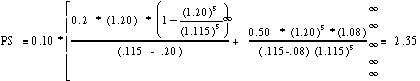For instance if the firm's profit margin declines from 10% to 5%, and the sales/BV remains unchanged:

New Growth rate in first five years = Retention Ratio * Profit Margin * Sales/BV= .8 * .05 * 2.50 = 10%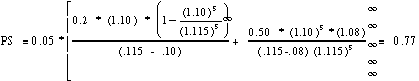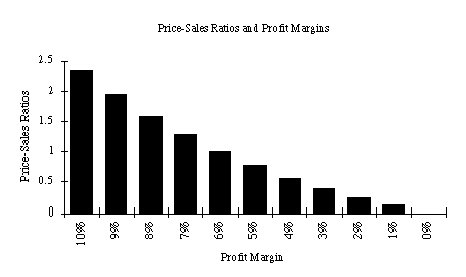Valuation Effects of changing from high-margin to low-margin strategy

Porter, in his examination of generic strategies, suggests that firms can follow one of two strategies to gain competitive advantage.

In the first, the firm offers a product similar to that offered by its competitors, but at a much lower price, and hopes to establish cost leadership.

In the second strategy, the firm attempts to differentiate its product from its competitors, and charge a price premium. The choice between a 'high margin, low volume' strategy (differentiation) and a 'low margin, high volume" (cost leadership) strategy can be examined using the price-sales multiple. This is illustrated in the following example.

Illustration 25 : Examining the effects of moving to a lower-margin, higher volume strategy: Philip Morris

Philip Morris had sales of \$59,131 million, earned \$4,939 million in net income and had a book value of equity of \$12,563 million in 1992. The firm paid 42% of its earnings as dividends in 1992. The beta for the stock was 1.10.

Based upon 1992 figures, the inputs for the price/sales ratio calculation would be:

Profit Margin = 8.35% Beta for the stock = 1.10

Sales / Book Value of Equity = 4.71 Expected Return = 7% + 1.1 (5.5%)

Book Value per share = \$14.10 = 13.05%

Retention Ratio = 58%

Expected growth rate over next five years = Retention Ratio * Profit Margin * Sales/Book

Value = 0.58 * 0.0835 * 4.71 = 22.80%

Expected growth rate after five years = 6% Expected payout ratio after five years = 65%

Price/Sales Ratio1992 Margins =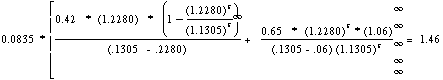Sales/Book Value1992 Margins = 4.71

In April 1993, Philip Morris announced that it was cutting prices on its Marlboro Brand of cigarettes because of increasing competition from low-priced competitors.

This was viewed by many analysts as a precursor of further price cuts and as a signal of a move to a lower-margin strategy. Assume that the profit margin will decline to 7% from 8.35%, as a consequence. All the other inputs are unchanged.

Expected growth rate over next five years = Retention Ratio * Profit Margin * Sales/Book Value = 0.58 * 0.07 * 4.71 = 19.12%

Expected growth rate after five years = 6% Expected payout ratio after five years = 65%

Price/Sales Ratio1992 Margins =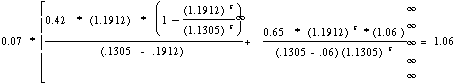As a consequence of the new lower-price strategy, the price-sales ratio will decline from 1.46 to 1.06. Unless the sales/book value ratio increases by an equivalent margin (27.40%), the value of Philip Morris will decrease. In the case where profit margins decline by this magnitude and the sales/book value is not expected to increase, the value will decline by 27.40%.

The market reacted negatively to the announcement of price cuts, and the stock price dropped approximately 20% on the announcement.

An aside on market share

There are some firms which have focused on maximizing market share as their primary objective function. The linkage between increased market share and market value is a tenuous one, and can be examined using the profit-margin/price-sales ratio framework suggested above. If increasing market share leads to higher margins, either because of economies of scale driving down costs, or increased market power driving out competitors, it will lead to higher value. If the increase in the market share is accompanied by lower prices and profit margins, the net effect on value can be negative.

From Net Income to Operating Income and Equity to Value

The profits margins for firms can be stated in terms of net income (as they have in all the examples so far) or in terms of operating income (EBIT). If pre-tax operating margins are used, the appropriate value estimate is that of the firm. In particular, if one makes the assumption that

Free Cash Flow to the Firm = EBIT (1 - tax rate): Net Capital exp. and working capital needs are zero.

Then the Value of the Firm can be written as a function of the after-tax operating margin= (EBIT (1-t)/Sales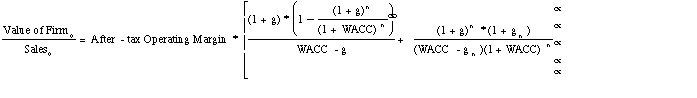where,

g = Growth rate in after-tax operating income for the first n years

gn = Growth rate in after-tax operating income after n years forever (Stable growth rate)

WACC = Weighted average cost of capital

The mismatch that should draw attention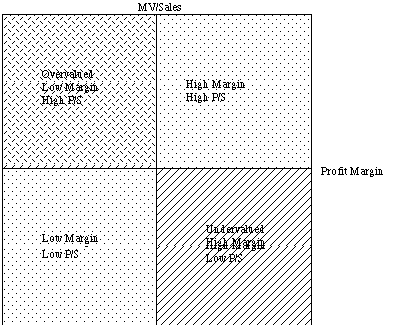Price-Sales Ratios and Cross-sectional Data

In this approach, the cross-sectional data is used to estimate the price to sales ratio as a function of fundamental variables - profit margin, dividend payout, beta and growth rate in earnings.

Price/Sales = a + b Payout + c Growth rate in earnings + d Beta + e Profit Margin

Crosssectional Regressions

Year Regression R squared

1987 PS = 0.7894 + .0008 PAYOUT - 0.2734 BETA+ 0.5022 EGR + 6.46 MARGIN 0.4434

1988 PS = 0.1660 + .0006 PAYOUT - 0.0692 BETA + 0.5504 EGR + 10.31 MARGIN 0.7856

1989 PS = 0.4911 + .0393 PAYOUT - 0.0282 BETA + 0.2836 EGR + 10.25 MARGIN 0.4601

1990 PS = 0.0826 + .0105 PAYOUT - 0.1073 BETA + 0.5449 EGR + 10.36 MARGIN 0.8885

1991 PS = 0.5189 + 0.2749 PAYOUT - 0.2485 BETA + 0.4948 EGR + 8.17 MARGIN 0.4853

PS = Price /Sales Ratio at the end of the year

MARGIN = Profit Margin for the year = Net Income / Sales for the year (in %)

PAYOUT = Payout Ratio = Dividends / Earnings BETA = Beta of the stock

EGR = Earnings Growth rate over the previous five years

1995: PS = -0.1967 + 0.4503 PAYOUT - 0.1769 BETA + 0.0820 EGR + 13.02 MARGIN

R squared=0.7726

EGR = Expected earnings growth rate over next five years

Illustration 27: Valuing an acquisition target using price-sales regressions

Sales in 1994 = 4350 million

Net Income in 1994 = 845 million

Beta = 0.60

Dividend Payout Ratio = 50%

First compute the variables in the desired units,

Profit Margin =845/4350 = 19.43%

Payout = 50%

Earnings Growth Rate= 10%

Beta = 0.60

Predicted Price/Sales Ratio = - 0.1967 + 0.4503 (0.50) - 0.1769 (0.60) + 0.0820 (0.10) + 13.02 (0.1943) = 2.46

Predicted Market Value of Equity = 2.46 * 4350 = \$10,701 million

Using price-sales ratios in investment strategies

• Senchack and Martin (1987) compared the performance of low price-sales ratio portfolios with low price-earnings ratio portfolios, and concluded that the low price-sales ratio portfolio outperformed the market but not the low price-earnings ratio portfolio. They also found that the low price-earnings ratio strategy earned more consistent returns than a low price-sales ratio strategy, and that a low price-sales ratio strategy was more biased towards picking smaller firms.
• Jacobs and Levy (1988a) tested the value of low price-sales ratios (standardized by the price-sales ratio of the industries in which the firms operated) as part of a general effort to disentangle the forces influencing equity returns. They concluded that low price-sales ratios, by themselves, yielded an excess return of 0.17% a month between 1978 and 1986, which was statistically significant. Even when other factors were thrown into the analysis, the price-sales ratios remained a significant factor in explaining excess returns (together with price-earnings ratio and size).
• When we created undervalued and overvalued portfolios (using price/sales ratios and profit margins) from 1981 to 1990, the undervalued portfolio outperformed the S&P 500 by about 6%.